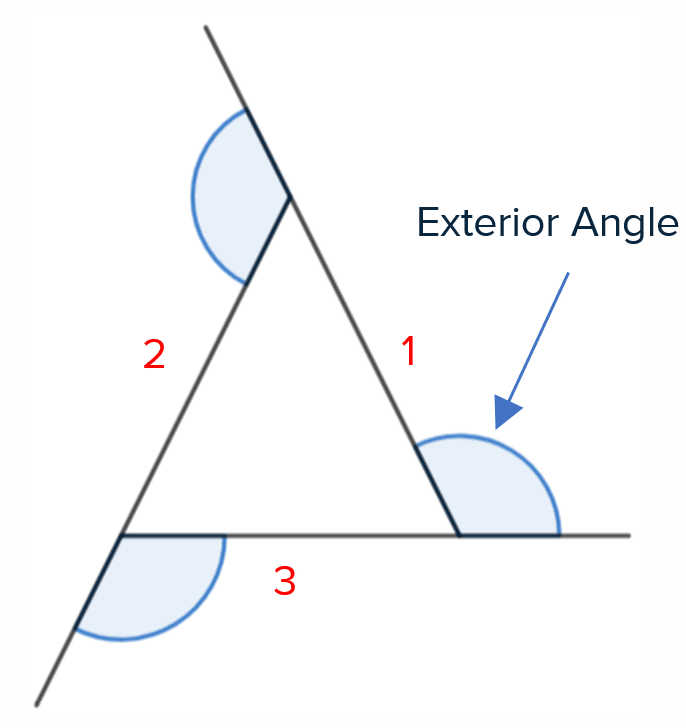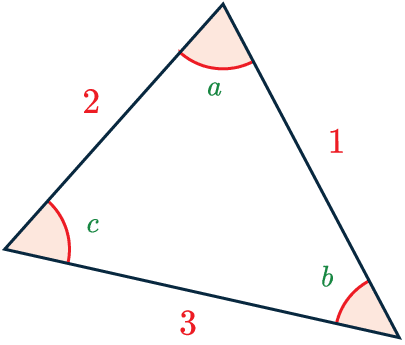# Interior and Exterior Angles

GCSEKS3Level 4-5AQAEdexcelEdexcel iGCSEOCRWJEC

## Interior and Exterior Angles

The interior angles of a shape are the angles inside the shape.

The exterior angles are the angles formed between a side-length and an extension.

Rule: Interior and exterior angles add up to $180\degree$.

Having the ability to rearrange equations will help with interior and exterior angle questions.

Level 4-5GCSEKS3AQAEdexcelOCRWJECEdexcel iGCSE

## Exterior Angles

Rule: The exterior angle = $\dfrac{360\degree}{\textcolor{red}{n}}$

where $\textcolor{red}{n}$ is the number of sides.

The sum of all the exterior angles will equal $360\degree$.For the triangle shown, we can see it has $\textcolor{red}{3}$ sides, so to calculate an exterior angle we do:

$\dfrac{360\degree}{\textcolor{red}{3}} = 120\degree$

Level 4-5GCSEKS3AQAEdexcelOCRWJECEdexcel iGCSE

## Interior Angles

Rule: Sum of interior angles = $(\textcolor{red}{n} - 2) \times 180\degree$

Where $\textcolor{red}{n}$ is the number of sides.To find the sum of the interior angles for the triangle shown we do the following:

$(\textcolor{red}{3} - 2) \times 180\degree = 180\degree$

This means that

$\textcolor{limegreen}{a} + \textcolor{limegreen}{b} + \textcolor{limegreen}{c} = 180\degree$

Note: You can find the interior angle of a regular polygon by dividing the sum of the angles by the number of angles. You can also find the exterior angle first then minus from $180\degree$ to get the interior angle.

Level 4-5GCSEKS3AQAEdexcelOCRWJECEdexcel iGCSE

## Example: Finding Interior and Exterior Angles

$ABCD$ is a quadrilateral.

Find the missing angle marked $x$.

[2 marks]This is a $4$-sided shape, to work out the interior angles we calculate the following:

$(\textcolor{red}{n}-2)\times 180 =360\degree$.

Next we can work out the size of $\angle CDB$ as angles on a straight line add up to $180\degree$.

$180 - 121 = 59\degree$

Now we know the other $3$ interior angles, we get that

$x = 360 - 84 - 100 - 59 = 117\degree$

Level 4-5GCSEKS3AQAEdexcelOCRWJECEdexcel iGCSE

## Interior and Exterior Angles Example Questions

This shape has 5 sides, so its interior angles add up to,

$180 \times (5 - 2) = 540\degree$

Hence each interior angle is,

$x\degree=540\degree \div 5 = 108\degree$Gold Standard Education

This shape has 8 sides, so its interior angles add up to,

$180 \times (8 - 2) = 1080\degree$

Hence each interior angle is,

$x\degree=1080\degree \div 8 = 135\degree$Gold Standard Education

This shape has 5 sides, so its interior angles must add up to

$180 \times (5 - 2) = 540\degree$.

We can’t find this solution with one calculation as we did previously, but we can express the statement “the interior angles add up to 540” as an equation. This looks like

$33 + 140 + 2x + x + (x + 75) = 540$

Now, this is a linear equation we can solve. Collecting like terms on the left-hand side, we get

$4x + 248 = 540$.

Subtract 248 from both sides to get

$4x = 292$.

Finally, divide by 4 to get the answer:

$x = 292 \div 4 = 73\degree$Gold Standard Education

This shape has 4 sides, so its interior angles add up to

$180 \times (4 - 2) = 360\degree$.

We don’t have any way of expression two of the interior angles at the moment, but we do have their associated exterior angles, and we know that interior plus exterior equals 180. So, we get

$\text{interior angle CDB } = 180 - (y + 48) = 132 - y$

Furthermore, we get

$\text{interior angle CAB } = 180 - 68 = 112$

Now we have figures/expressions for each interior angle, so we write the sum of them equal to 360 in equation form:

$112 + 90 + 2y + (132 - y) = 360$

Collecting like terms on the left-hand side, we get

$y + 334 = 360$

Then, if we subtract 334 from both sides we get the answer to be

$y = 360 - 334 = 26\degree$.Gold Standard Education

## Interior and Exterior Angles Worksheet and Example Questions

### (NEW) Interior and Exterior Angles Exam Style Questions - MME

Level 4-5GCSENewOfficial MME

## Interior and Exterior Angles Drill Questions

### Interior angles of polygons

Level 4-5GCSEGold Standard Education